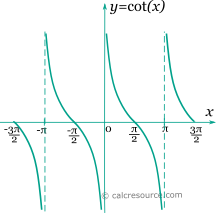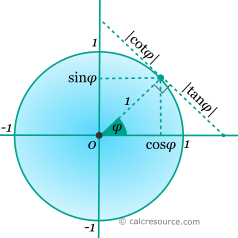Jump to
Table of Contents
Share this

## Trigonometric cotangent calculator

This tool evaluates the cotangent of a number: cot(x). Enter the argument x, in radians or degrees, below.

 x = deg rad Result: cot(x) = Also, at same x: sin(x) = cos(x) = tan(x) =Table of Contents
Share this

## Definitions

### General

The cotangent function, in modern notation written as cot(x), is a trigonometric function. Trigonometric functions are commonly established as functions of angle, in the context of right triangle geometry. This way, the cotangent of an angle φ is defined as the ratio of the adjacent side of a right triangle containing φ, divided by the opposite side (see figure):Unfortunately the above definition is limited to a range of angles between 0 and 90°. Extending that range, a convenient definition employs a unit circle (radius equal to 1). Any point of the circle, corresponds to a pair of sine and cosine values, of the angle that is contained by the horizontal positive axis and the radial segment towards that point, as shown in the figure below. The absolute value of cotangent is represented by the length of the tangent segment from the circle point towards the vertical axis. The angle φ is assumed positive in counter-clockwise direction. Angles larger than 90°, as well as negative ones, are possible, if the appropriate sign of the coordinates is respected.The above definitions of cotangent function are based on a geometrical construct (right triangle or unit circle) and assume that its argument is an angle. This association to an angle is restrictive, considering the broad use of trigonometric functions in mathematics, physics, engineering etc. Applications may accept any real value as argument, with any imaginable meaning given to it, or no meaning at all. In that case, x should be better measured in radians (). Thus, derivation and integration rules are more conveniently applied.

### Series

All trigonometric functions can be defined in an infinite series form. Cotangent function can be written as:

The above series converges for . Bndenotes the n-th Bernulli number.

### Properties

The cotangent function is periodic, with a period equal to π. Therefore:

Cotangent is associated with sine and cosine and tan functions with the formulas:

The derivative of the cotangent function (x in radians) is given by the following expressions:

The integral of the cotangent function (x in radians) is given by: